# ACT Mathematics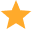|

20 tests 400 questions

### What we offer

Personal performance system to track your progress

Preparation for CEB Cubiks, Kenexa, Saville, and more!

Detailed instructions and worked solutions

ACT Mathematics|

20 tests 400 questions

## What is the Kaplan ACT Mathematics test?

The ACT, short for American College Testing, is the leading US admissions test, measuring what you learn in high school to determine your academic readiness for college. The ACT consists of the sections English, Math, Reading and Science – and an optional section focusing on writing.

This preparation pack will help you get prepared for the hardest section of the ACT, the math test! The math part of the ACT test is a 60-minute, 60-question math test and calculators are permitted in this section only.

Assessment-Training.com offers a tailor-made package of tests especially made to mimic the ACT Math test. Start your training now to make sure that you’re fully prepared for your ACT!

The ACT Math Package consists of:

• Numerical Skills/Pre-algebra Tests
• Algebra Tests
• College Algebra Tests
• Geometry Tests
• Trigonometry Tests

All of our practice tests come with extensive explanations and a description of how the questions can be solved. Furthermore, our Personal Progression System will help you track your progress and give a detailed view of your performance. This system will help you identify your stronger and weaker points and provides insight into your areas of development. The progression tool keeps track of your scores, test times and overall progress.

Start training now and get immediate access to the tests and practice 24/7 on your computer, phone or tablet!

## Numerical skills/Pre-algebra Placement Test

The questions in this part of the ACT math test range in content from basic arithmetic concepts and skills to the knowledge and skills considered prerequisites for a first algebra course. The Numerical Skills/Pre-algebra placement test includes items from more than a dozen content areas; however, a majority of the questions come from the following 7 categories:

1. Operations with integers
2. Operations with fractions
3. Operations with decimals
4. Positive Integer exponents, square roots, and Scientific Notation
5. Ratios and Proportions
6. Percentages
7. Averages (Means, Medians, and Modes)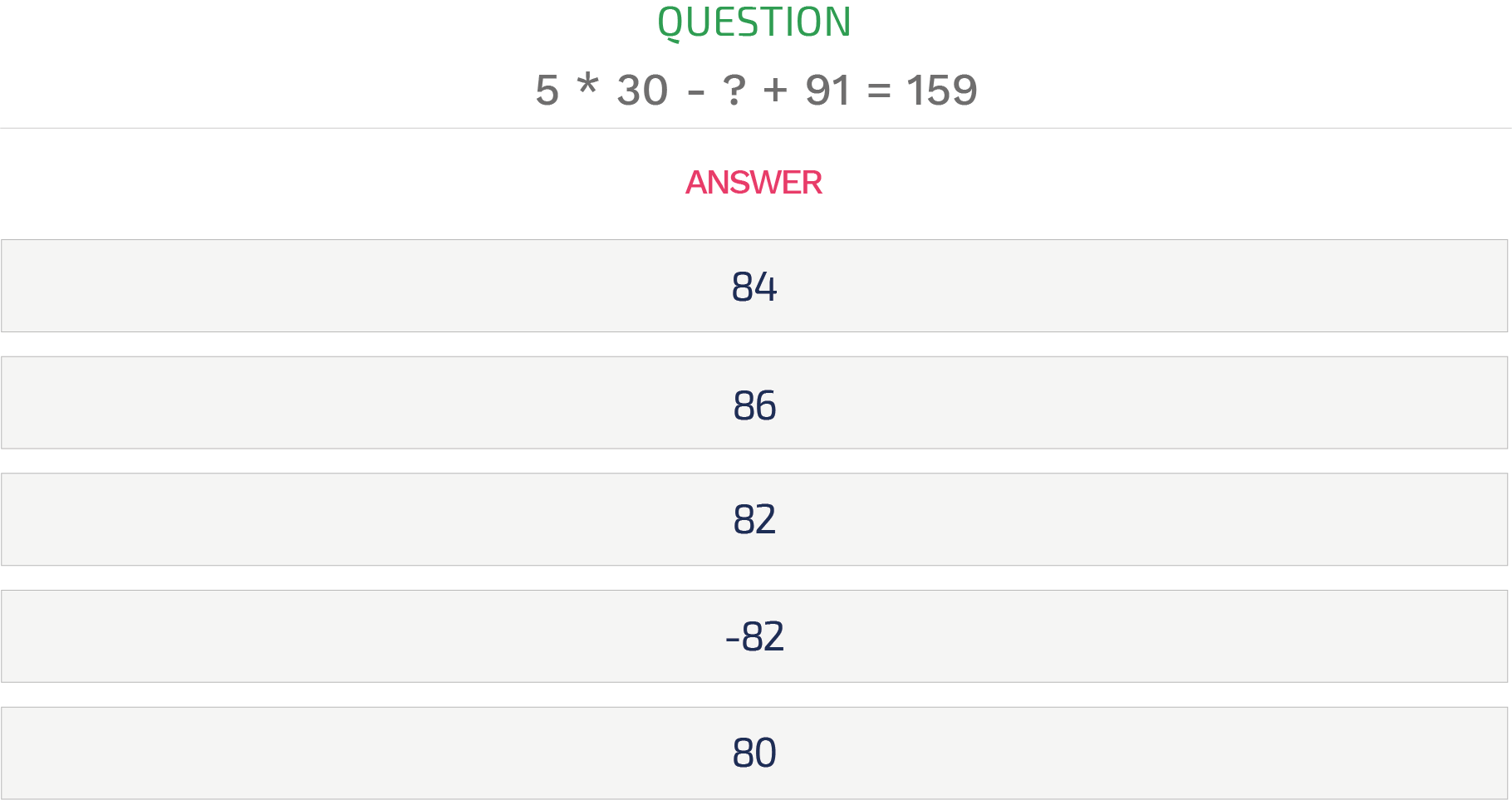Example Numerical skills/Pre-algebra question, click here or on the picture for a free test.

Explanation:

Let the unknown number be x, then

150 - x = 159 - 91 = 68

x = 150 - 68 = 82

The right answer is C, 82.

## Algebra Placement Test

The questions in this part of the ACT math test are composed of items from three curricular areas, being elementary algebra, coordinate geometry, and intermediate algebra. Each of these three categories is further subdivided into a number of more specific content areas. The Algebra Placement Test includes more than 20 content areas; however, a majority of the questions are from the following 8 categories:

1. Substituting values into algebraic expressions
2. Setting up equations for given situations
3. Basic operations with polynomials
4. Factoring polynomials
5. Linear equations in one variable
6. Exponents and radicals
7. Rational expressions
8. Linear equations in two variables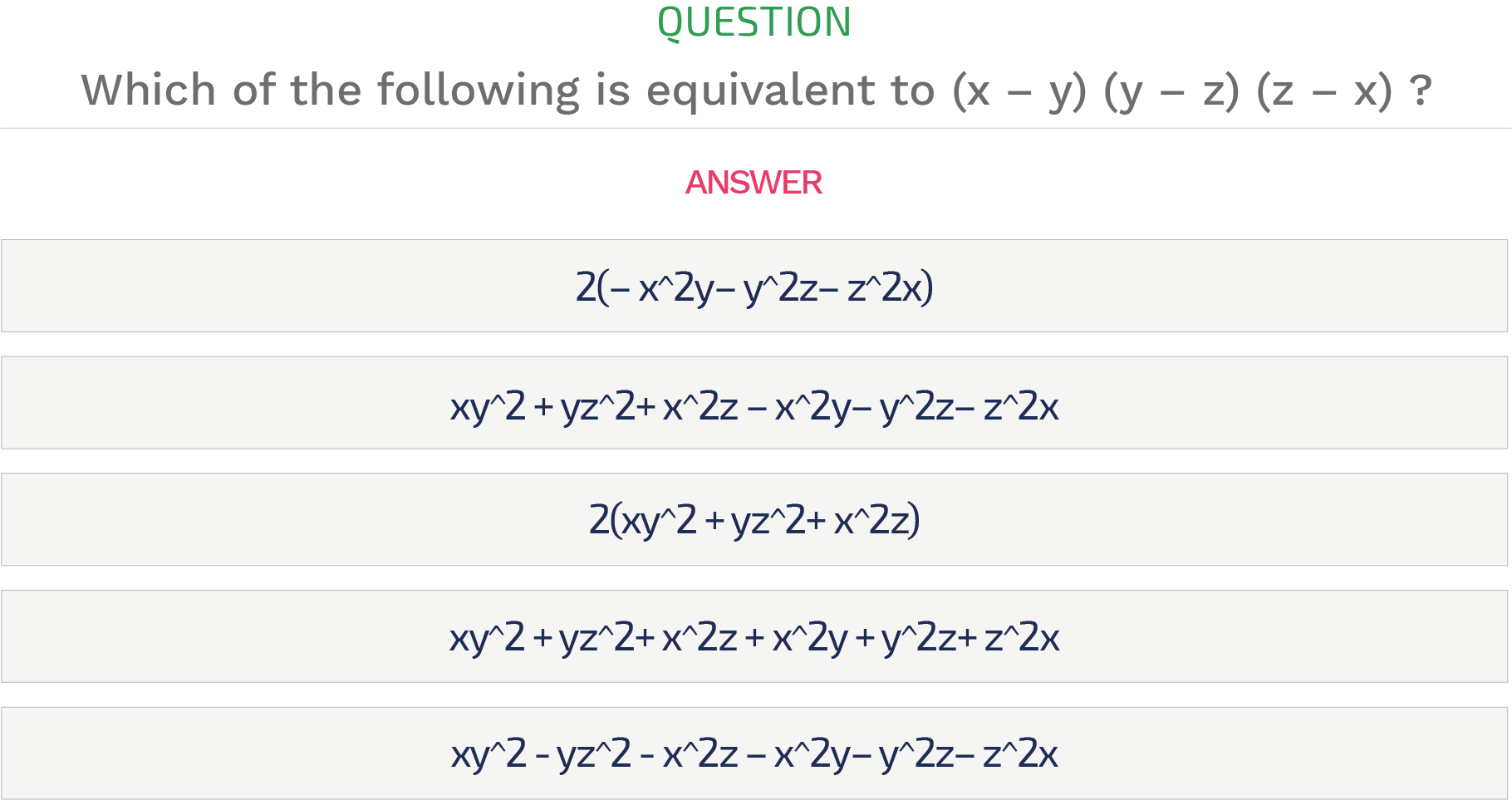For example Algebra question, click here or on the picture for a free test.

Explanation:

The correct answer is B

Multiplying step by step:
Here, (x – y) (y – z) (z – x)
= (xy – xz – y^2 + yz) (z – x)
= (xyz – z^2 x– y^2z + yz^2 – x^2y + x^2z + xy^2 –xyz)
= xy^2 + yz^2+ x^2z – x^2y– y^2z– z^2x
Therefore, the answer is B.

## College Algebra Placement Test

The questions in this part of the ACT math test focus on algebra knowledge and skills in a variety of content areas. The majority of items come from the following 5 categories:

1. Functions
2. Exponents
3. Complex numbers
4. Arithmetic and geometric sequences and series
5. Matrices (basic operations, equations, and determinants)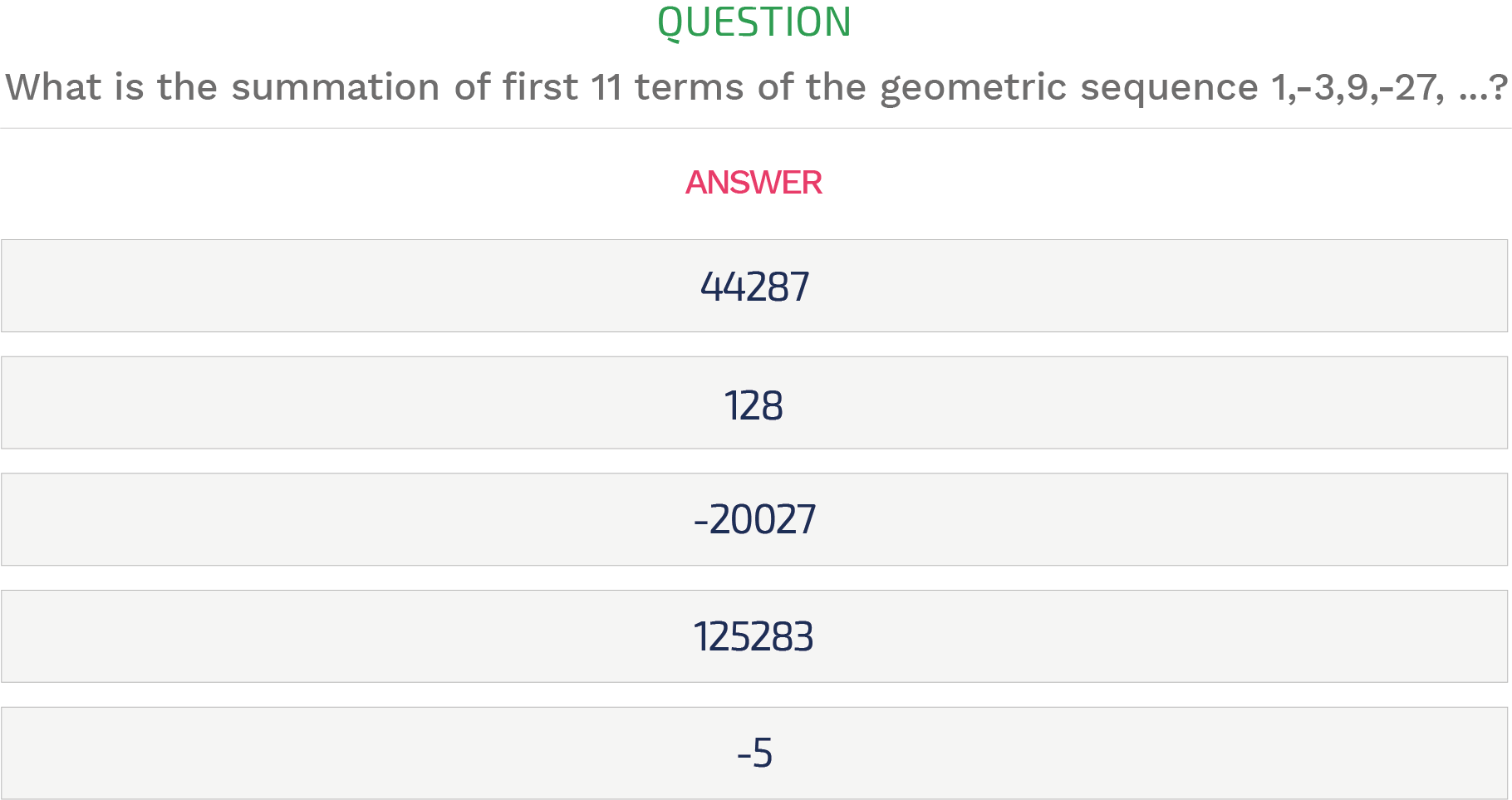Example College algebra question, click here or on the picture for a free test.

Explanation:

Here, 1st term: a=1
Common ratio, r= (-3)/1=-3
Number of terms, n=11
The summation, Sn=(a(1-r^n))/(1-r)
S11 = (1(1-(-3)^11))/(1-(-3))
=44287
Therefore, the answer is A.

## Geometry Placement Test

The questions in this part of the ACT math test focus on the following content areas:

1. Triangles (perimeter, area, etc.)
2. Circles (perimeter, area, arcs, etc.)
3. Angles (supplementary, complementary, adjacent, vertical, etc.)
4. Rectangles (perimeter, area, etc.)
5. Three-dimensional concepts
6. Hybrid (composite) shapes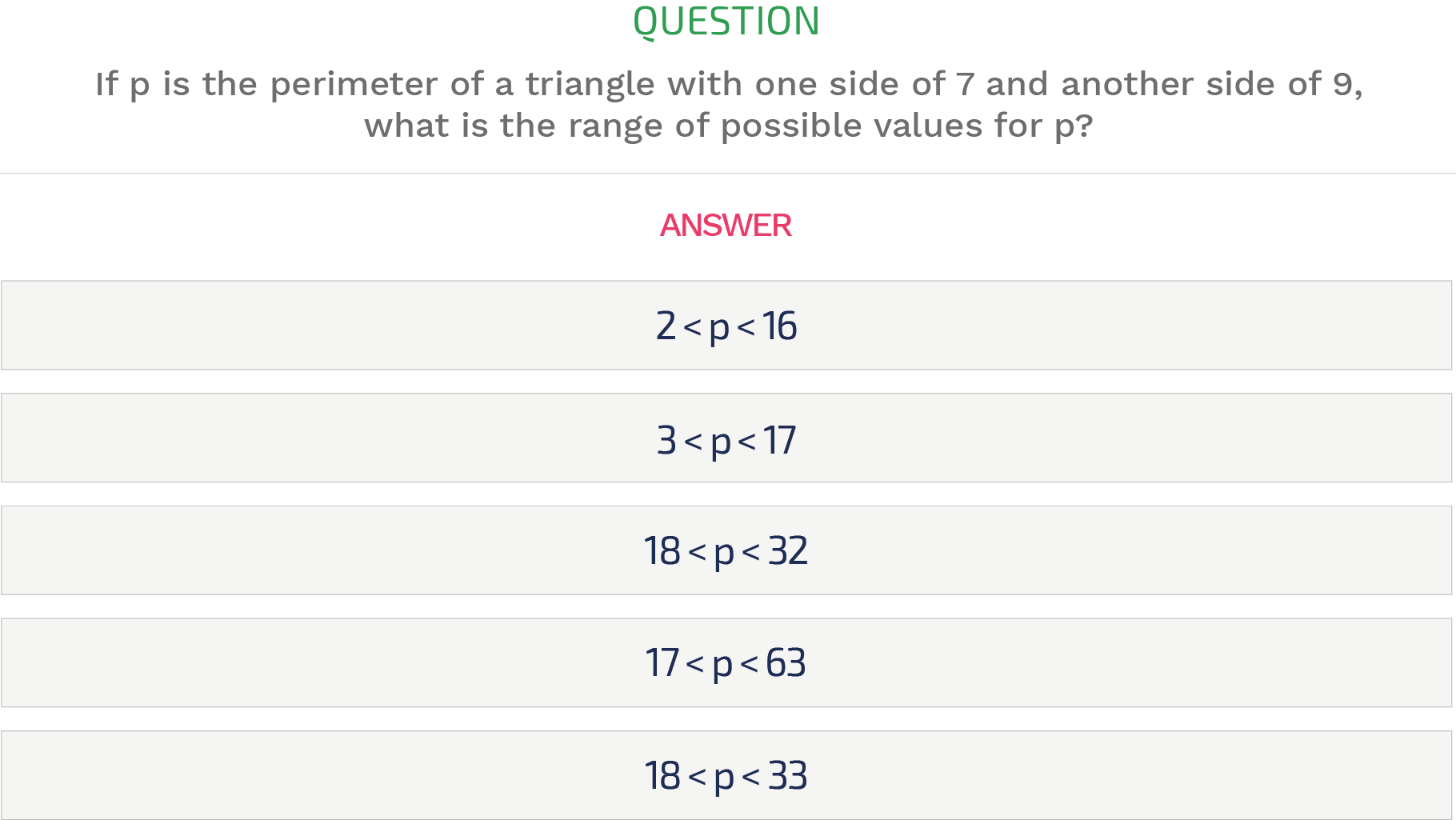For example Geometry question, click here or on the picture for a free test.

Explanation:

We know, the third side of a triangle is less than the sum of the other two.
So, the third side should be less than (7+ 9) =16.
So, the perimeter should be less than 7+ 9+ 16= 32
Again, the third side should be greater than the subtraction of the other two.
So, the third side should be greater than (9 – 7) =2
So, the perimeter should be greater than 7+ 9+ 2= 18
Therefore, the right answer is C.

## Trigonometry Placement Test

The questions in this part of the ACT math test focus on the following content areas:

1. Trigonometric functions and identities
2. Right-triangle trigonometry
3. Trigonometric equations and inequalities
4. Graphs of trigonometric functions
5. Special angles (multiples of 30 and 45 degrees)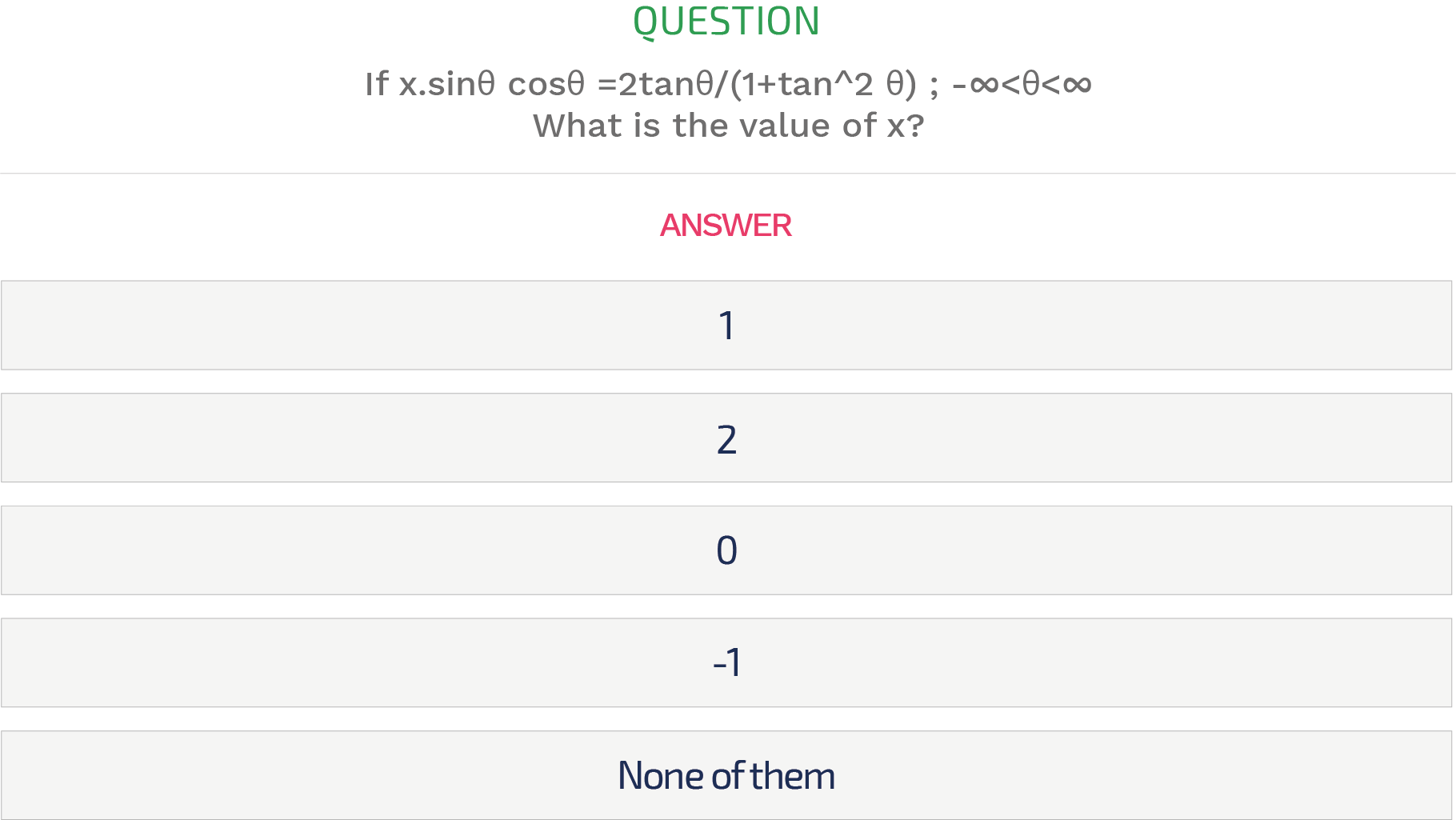For example Trigonometry question, click here or on the picture for a free test.

Explanation:

2tanθ/(1+tan^2 θ⁡)=(2 sinθ/cosθ)/(1+(sin^2 θ)/(cos^2 θ))=(2 sinθ cosθ)/(cos^2 θ+sin^2 θ)=2 sinθ cosθ
Comparing with x.sinθ cosθ ,we get x=2
Therefore, the right answer is B.

## Tips and Tricks to ace your ACT Mathematics test

Doing some research about the test that you’re expecting is the most important step in preparation for any aptitude test. If you know what to expect you will increase your chances of performing well due to the fact that you’re familiar with the formats. Also, by practising aptitude tests you will reveal your own strengths and weaknesses that you can work on in preparation for the actual assessment.

These effective test-taking strategy tips and tricks are well worth remembering before you take the actual ACT Math test:

• Practice, practice and practice some more to familiarize yourself with the content areas of the test.
• Read the question and instructions properly to make sure you understand the type of answer required. Even though this seems logical, often candidates miss out on very important information. Multiple-choice tests use so-called ‘distractors’ as answer possibilities. These distractors are answers options that are deliberately similar to the right answer; in other words, the answer possibilities are designed to test if you read the question and provided information property.
• Make sure you learn how to efficiently use a calculator properly and wisely. Effective use of a calculator can help you save precious time and energy during the real test.
• Do not linger over problems that take too much time. Try to solve as many as you can; then return to the others in the time you have left for the test.
• In general, unless otherwise stated, all of the following should be assumed during the ACT Math test:
• Illustrative figures are not necessarily drawn to scale.
• Geometric figures lie in a plane.
• The word line indicates a straight line.
• The word average indicates the arithmetic mean.

## Why practice?

Practice makes perfect! You will see that through practise your performance will improve. Familiarizing yourself with psychometric tests is the first step to success. Through practice, you will get a clear picture of what these tests are, what they look like, what they measure, and what the testing conditions will be.

Assessment-Training.com is the go-to platform for certification practise, assessment/aptitude test training, and job application preparation. We offer tailor-made preparation packages with tests in the same style as the actual ACT Mathematics assessment.

In addition to the fact that all our practice tests come with worked solutions and an explanation on how to get to the right answer, we offer you a unique Personal Progression Tracking System.

This Progression system will help you track your progress and give a detailed view of your performances. It will help you identify your stronger and weaker points and gives insight into your areas of development. The progression tool keeps track of your scores, test times and overall progression.

We offer you the tools to reduce anxiety and make sure you get the most out of yourself on the day of your certification, assessment test or job application interview! Research among our users showed that through practise your scores can improve up to 80%.

Over 96% of our customers would recommend Assessment-Training for practice.

Start training now and get immediate access to the tests and practice 24/7 on your computer, phone or tablet!

Assessment-Training.com is the market leader in online assessment preparation. With our continuous research and development, we provide a leading-edge e-learning platform. We keep improving our tests to deliver tests with the same standards as the latest tests of all the big assessment publishers. Our products help candidates complete their application fully prepared. Our goal is to help you get ready for your assessment, regardless of the company, job, or level you are applying for!

To help you ace your ACT Mathematics Assessment we put together a preparation package, tailor-made for ACT Mathematics Assessment. These tests contain the same questions you can expect in your actual ACT Mathematics Assessment. The ACT Mathematics Preparation Package consists of the following tests:

• Numerical Skills/Pre-algebra Tests
• Algebra Tests
• College Algebra Tests
• Geometry Tests
• Trigonometry Tests

All practice tests come with worked solutions and an explanation on how to get to the right answer. Our Personal Progression System will help you track your progress and give a detailed view of your performance. Assessment-Training is more than just a training platform, we are here to help you!

Start training now and get immediate access to the tests and practice 24/7 on your computer, phone or tablet!

# Improve your hiring chances by 81%.

## Detailed instructions and worked solutions for every question. Prepare to succeed!

One-time payment. No recurring fees!

## ACT Mathematics Preparation Package

Our tailor-made ACT Mathematics test preparation package helps you to adjust to the question styles and difficulty levels of the actual test.
• 20 Tests
• 400 Questions
• One-off payment